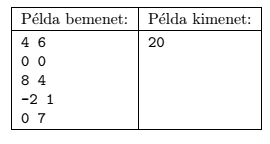Mathematical and Physical Journal
for High Schools
Issued by the MATFUND Foundation
 Already signed up? New to KöMaL?

#Problem S. 100. (September 2015)

S. 100. We are given $\displaystyle N$ squares ($\displaystyle 1\le N\le 300\;000$) with sides parallel to the coordinate axes. The size of each square is $\displaystyle K\times K$ ($\displaystyle 1\le K\le 1\;000\;000$), and the center of each square is also given by a pair $\displaystyle (x; y)$ ($\displaystyle -1\;000\;000\le x, y\le 1\;000\;000$). Some squares do not overlap, but sometimes one or more pairs of squares overlap with an intersection having positive area.

Your program should read $\displaystyle N$ and $\displaystyle K$ from the first line of the standard input, then the $\displaystyle x_i$, $\displaystyle y_i$ coordinates from the following $\displaystyle N$ lines. The first and only line of the standard output should be either 0 if no two squares overlap, or -1 if there are more than one overlapping pairs, or the area of the intersection if there is exactly one pair of overlapping squares.

In the example, Példa bemenet'' is a sample input, while Példa kimenet'' is the corresponding output. Explanation: squares 1 and 3 overlap.Scoring and bounds. You can get 1 point for a brief and proper documentation clearly describing your solution. Nine further points can be obtained provided that your program solves any valid input within 1 second of running time.

The source code of your program with a short documentation-also describing which developer environment to use for compiling the source-should be submitted in a compressed file s100.zip.

(10 pont)

Deadline expired on October 12, 2015.

### Statistics:

 25 students sent a solution. 10 points: Alexy Marcell, Dóczi András, Erdős Márton, Gáspár Attila, Mernyei Péter, Nagy Nándor, Noszály Áron, Zalavári Márton, Zarándy Álmos. 8 points: 3 students. 7 points: 2 students. 5 points: 2 students. 4 points: 1 student. 3 points: 3 students. 1 point: 1 student. 0 point: 4 students.

Problems in Information Technology of KöMaL, September 2015